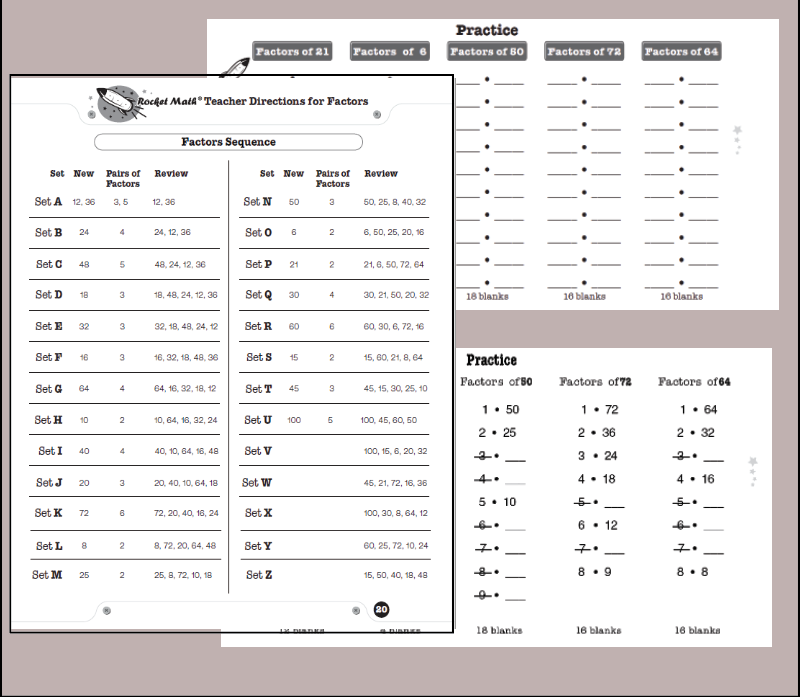## What do students learn?

Students learn a systematic way of finding factor pairs, which results in being able to memorize the factor pairs of common numbers.

What are the factor pairs of 24? Answer: 1 and 24, 2 and 12, 3 and 8, 4 and 6. This is what students learn by memory.

By following our systematic “How to Factor” procedure, students are certain to find and remember all the factor pairs of common numbers.

Finding the greatest common factor and simplifying fractions will be a breeze after students learn this easy method and practice finding all the factors of these common numbers. Students learn all the factor pairs for these numbers in this sequence: 12, 36, 24, 48, 18, 32, 16, 64, 10, 40, 20, 72, 8, 25, 50, 6, 21, 30, 60, 15, 45, and 100.

## Video Lesson on “How to Factor” for students

Click here to see the critical “How to Factor” online video lesson from Dr. Don.

Or paste this into your browser so students can watch the lesson:  https://www.educreations.com/lesson/view/how-to-find-all-the-factors-of-a-number/46790401/?ref=link

## How students use this Learning Track

Students practice with a partner, take a daily one-minute timing, fill in a Rocket Chart, just like regular Rocket Math. There are also special directions for the things that are different in the Factors Learning Track.

Be sure to give the special Writing Speed Test for Factors. It’s found in the virtual filing cabinet drawer. It gives the student special goals for writing factor pairs.